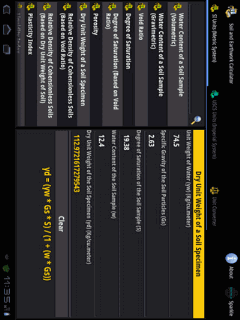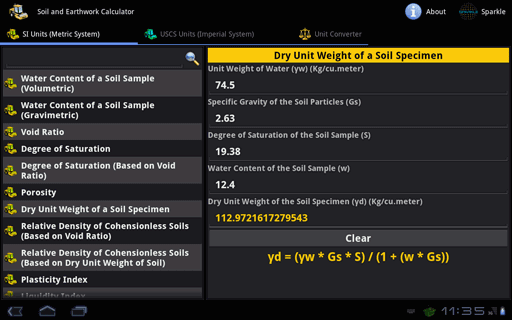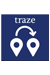### Soil + Earthwork Calculator for Android Tablet##### Summary:
92 Calculators & Converters related to Soil, Earthwork & Civil Engineering. Available in both Metric & Imperial Units with Formulas. Automatic Calculations & Accurate Conversions.
1.0
Android
##### Category:
Education,Calculator
\$2.99
##### Requiements:
Works with all Android Tablet
##### Overview

Soil and Earthwork Calculator contains 92 Calculators and Converters, that can quickly and easily calculate and convert different Soil, Earthwork and Civil Engineering parameters. Automatic & Accurate Calculations and Conversions with every Unit and Value Changes. Available in Imperial and Metric Units. Most Comprehensive Soil and Earthwork Calculator.Available in Metric and Imperial Units

Soil and Earthwork Calculator contains following 60 Calculators:

• Water Content of a Soil Sample (Volumetric)
• Water Content of a Soil Sample (Gravimetric)
• Void Ratio
• Degree of Saturation
• Degree of Saturation (Based on Void Ratio)
• Porosity
• Dry Unit Weight of a Soil Specimen

• Relative Density of Cohensionless Soils (Based on Void Ratio)
• Relative Density of Cohensionless Soils (Based on Dry Unit Weight of Soil)
• Plasticity Index
• Liquidity Index
• Shrinkage Index
• Activity

• Saturated Unit Weight of Soil
• Dry Unit Weight of Soil
• Buoyant Unit Weight of Soil

• Coefficient of Internal Friction of Soil
• Vertical Stress in Soil (Boussinesq Equation)
• Vertical Stress in Soil (Westergaard Equation)
• Coefficient of Active Pressure
• Coefficient of Passive Pressure

• Total Thrust from the Soil (Walls retaining Cohensionless Soils)
• Total Thrust from the Soil (Walls retaining Cohensionless Soils & Surface behind the Wall is Level)
• Total Thrust from the Soil (Walls retaining Cohensionless Soils and are Completely Restrained)
• Total Thrust from the Soil (Walls retaining Cohensionless Soils and are Completely Restrained & Surface behind the Wall is Level)
• Total Thrust from the Soil (Walls retaining Cohesive Soils and Free to Move a Considerable Amount)
• Total Thrust from the Soil (Walls retaining Cohesive Soils and Free to Move a Small Amount)
• Total Thrust from the Soil (Highly Cohesive Soils)
• Total Thrust from Water retained behind a Wall

• Effect of Surcharge on a Wall retaining Cohensionless Soil/Unsaturated Cohesive Soil
• Stability Number (Slope - Without Seepage Water)
• Stability Number (Slope - Seepage of Water)

• Ultimate Bearing Capacity of Soil (Prandtl's Equation)
• Relationship between Loads on Foundations and Settlement
• Volume of Soil
• Percentage of Moisture Content in Soil
• Field Density of Soil
• Dry Density of Soil
• Percentage of Compaction of Soil

• California Bearing Ratio
• Flow Rate
• Compaction Production

• Rolling Resistance (Earth Moving)
• Total Road Resistance/Total Pull (Earth Moving)
• Volume of Soil after Excavation
• Volume of Soil after Compaction

• Scraper Production
• Trips per Hour (Scraper)
• Amount of Material carried by a Machine
• Production Required
• Number of Scrapers needed on a Job
• Number of Scrapers a Pusher can Load
• Variable Time

• Wavelength of Vibration during Explosion
• Velocity of Particles distributed by Vibration during Explosion
• Acceleration of Particles distributed by Vibration during Explosion
• Overpressure due to Explosion
• Sound Pressure Level
Soil and Earthwork Calculator contains following 32 Converters:

• Acceleration
• Angle
• Area
• Density
• Energy/Work
• Flow Rate (Mass)
• Flow Rate (Volume)
• Fluid
• Force
• Frequency
• Hardness
• Length
• Mass
• Metric Weight
• Metrology
• Moment of Force
• Moment of Inertia
• Prefixes
• Pressure
• Specific Heat Capacity
• Specific Volume
• Temperature
• Thermal Conductivity
• Thermal Expansion
• Time
• Torque
• Velocity
• Viscosity (Dynamic)
• Viscosity (Oil & Water)
• Viscosity (Kinematic)
• Volume
Key Features:
• Complete coverage of calculators and converters in Soil, Earthwork and Civil Engineering Parameters.
• Automatic Calculation & Conversion of the Output with respect to changes in the Input/Options/Units.
• Formulas are provided for each calculator.
• Values of Higher Order can also be calculated.
• Extremely Accurate Calculations and Conversions.
• Professionally and Newly designed user-interface that speeds up Data Entry, Easy Viewing and Calculation Speed.
Most Comprehensive Soil and Earthwork Calculator

•  Traze - reach ur friendsShare location with friends & view theirs. Navigate & make calls, all in one go. Traze enables two-way communication. Its never been easier to reach someone. More...Civil Engineering Pack for Android Tablet574 Calculators & Converters related to Civil, Beams, Columns, Piling, Concrete, Survey, Soil & Earthwork, Structural Engineering, Bridges, Highway & Road, Hydraulics and Timber. More...